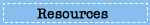# Energy Transfer Response Page

In this 20-minute task, students investigate which metal would be better for making the bottom of a cooking pan. While designing and conducting their investigations, students use a simulated calorimeter to test the specific heat capacities of two metals that could be used for the bottom of the pan.

Below are the questions, your answers, and the scoring criteria for each of the questions in this task. Use the navigation on the left to return to browsing the site or to take another task.

# Question 1

On the left is a graph showing the results when a 250 g block of copper at 60.0 degrees Celsius was placed in 250 g of water at 20.0 degrees Celsius.

Based on the data, what happened to the temperatures of the materials? Select all that apply.

A. The temperature of the water increased.
B. The temperature of the water decreased.
C. The temperature of the copper increased.
D. The temperature of the copper decreased.

Explain why these changes in temperature happened. In your answer, describe what happened to the water molecules and the copper atoms during these changes.

Sample Complete Student Response:

As time progressed the copper transferred a small portion of its heat to the water, providing the increase in water temperature and the decrease in the temperature of the copper. The water molecules would then speed up as the temperature rises. The atoms in the copper would slow down as the temperature decreases.

Complete:
Student response selects (A) The temperature of the water increased, and (D) The temperature of the copper decreased, and provides a correct explanation that consists of two parts:

• Indicates that when objects of different temperatures are in contact, thermal energy (heat) flows from the object at a higher temperature to the object at a lower temperature.
• Indicates that the motion (speed, number of collisions) of water molecules increases and the motion of copper atoms decreases.

Essential:
Student response selects (A) or (D), and no other option, and provides a correct explanation.

OR

Student response selects (A) and (D), and addresses one part of a correct explanation.

Partial:
Student response selects either (A) or (D) or a mixture of correct and incorrect options, and provides an explanation that addresses one part of a correct explanation.

OR

Student response selects (A) and (D), and provides no explanation or a general explanation related to the specific heat capacity or the temperature of the materials that does not include any incorrect information.

Unsatisfactory/Incorrect:
Student response is inadequate or incorrect.

Percentage of twelfth-grade students in each response category: 2009
Complete Essential Partial Unsatisfactory/Incorrect Omitted
4 23 11 61 #
# Rounds to zero.
NOTE: Detail may not sum to totals because of rounding.

# Question 2

You can now adjust the temperature and mass of both the copper and the water.

Run the simulation with 250 g of copper at 20.0 degrees Celsius and 250 g of water at 60.0 degrees Celsius.

Based on your data, which statement is true?

A. Copper had a greater change in temperature than water did.
B. Water had a greater change in temperature than copper did.
C. The temperatures of both copper and water changed the same amount.
D. The temperatures of both copper and water remained unchanged.

Percentage of twelfth-grade students in each response category: 2009
Choice A Choice B Choice C Choice D Omitted
77 7 8 7 1

# Question 3

Use the simulation to investigate whether copper or water has a higher specific heat capacity.

Based on your data, which substance has a higher specific heat capacity?

A. Copper
B. Water

Explain how you know. Use your data to support your explanation.

Sample Complete Student Response:

Water has a higher specific heat capacity because it has less of a tendency to change in temperature. What this signifies is that more energy is required to increase the temperature of the water. Copper with the temperature of 60 degrees Celsius, and the same mass as that of the water in which it was placed, cooled off to 23.4 degrees Celsius. On the other hand, the water, starting off with the temperature of 20 degrees Celsius, rose to only 23.4 degrees Celsius.

Score & Description:

Sample Data From Simulation: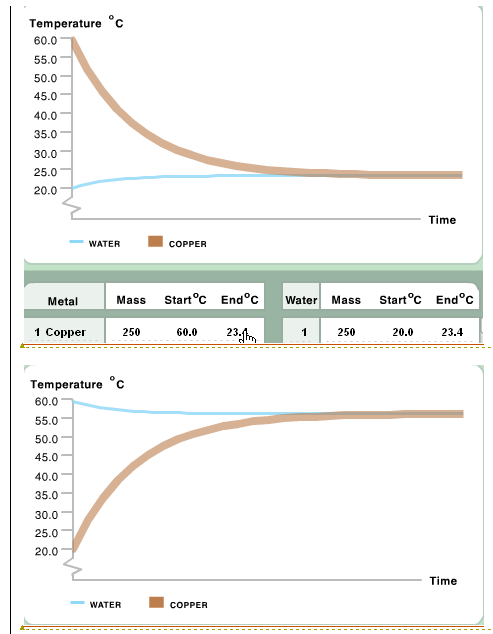Complete:
Student response selects (B) Water, and provides a correct explanation that includes a comparison of the amount of thermal energy transferred to change the temperature of (equal masses) of water and copper (1°C), and refers to valid data for support. Explanation indicates that the temperature of the water changed less than the temperature of copper (when equal masses of copper and water at different temperatures were placed in contact), which shows that more thermal energy (heat) is transferred per degree change in temperature of water than copper.

Essential:
Student response selects (B), and indicates that the temperature of the water changed less than the temperature of (an equal mass) the copper or that more thermal energy (heat) is transferred to change the temperature of (equal masses) of water (1°C) than copper (1°C).

Partial:
Student response selects (B), and provides a general observation based on valid data or provides some relevant data, but does not explain how the data were used to compare the specific heat capacities of the materials.

OR

Student response selects (A) Copper, but provides an explanation indicating that the temperature of the water changed less than the temperature of the copper, and provides valid data supporting correct choice (B).

Unsatisfactory/Incorrect:
Student response is inadequate or incorrect.

Percentage of twelfth-grade students in each response category: 2009
Complete Essential Partial Unsatisfactory/Incorrect Omitted
6 9 10 75 #
# Rounds to zero.
NOTE: Detail may not sum to totals because of rounding.

# Question 4

Describe the procedure you used to compare the specific heat capacities of the metals using the simulated calorimeter.

Describe how you decided which combinations of temperature and mass to set for each metal in the trials you ran.

Sample Complete Student Response:

The procedure used to compare the specific heat capacities of the metals was that I set the starting temperature of water at 60 degrees Celsius and the mass at 250 g. Then I set both metals mass's at 250 g and the temperature for both at 20 degrees Celsius. This allowed me to run the experiment and compare both metals using the same conditions. I decided to use 250 grams for both metals and water to use the most amount of metal and using the least amount of water. Setting the temperatures of both metals at 20 degrees Celsius allowed me to see by how many degrees each metal increased in temperature.

#### Scoring Guide

Complete:
Student describes a valid procedure that consists of three parts:

• Indicates that a minimum of two controlled trials, one for each metal, with the initial conditions (mass of metals, mass of water, initial metal temperature, and initial water temperature) held constant (the same for the two trials).
• Indicates that the initial temperature of the metals is at least 10°C different (higher, lower) from the initial temperature of the water (so that a change in temperature can be observed).
• observes the change in temperature of the metals and water.

Essential:
Student response addresses two parts of a valid procedure.

Partial:
Student response addresses one part of a valid procedure.

Unsatisfactory/Incorrect:
Student response is inadequate or incorrect.

Percentage of twelfth-grade students in each response category: 2009
Complete Essential Partial Unsatisfactory/Incorrect Omitted
5 21 26 40 7
NOTE: Detail may not sum to totals because of rounding.

# Question 5

Based on the data from your investigations, which metal has the higher specific heat capacity?

A. Copper
B. Aluminum

Explain how you know. Use your data to support your explanation.

Sample Complete Student Response:

The aluminum's temperature changed less than the copper's did under the same conditions. Thus, the aluminum must require more energy to change its temperature. Therefore, aluminum has the higher specific heat.

#### Scoring Guide

Valid data from simulation: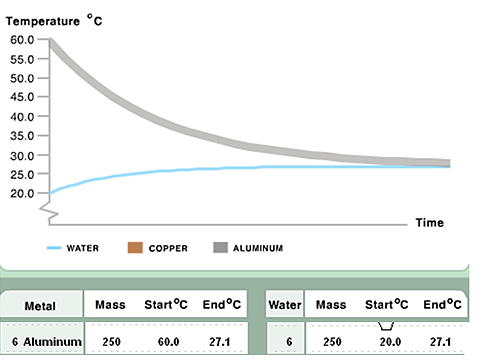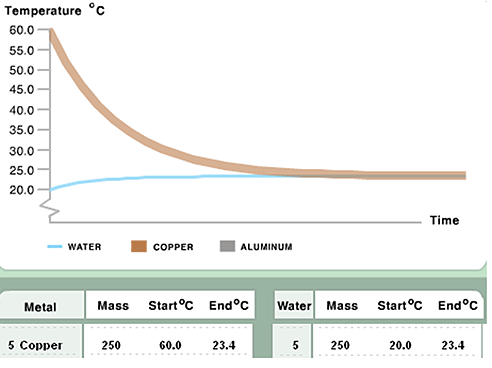Complete:
Student response selects (B) Aluminum, and provides an explanation demonstrating understanding that more thermal energy is required to change the temperature of aluminum compared to (the same mass of) copper, which is based on valid data. The data indicate that the temperature of the aluminum changed less (more slowly) than the temperature of the copper (or the temperature of the water changed more with aluminum than with copper).

Essential:
Student response selects (B), and compares the temperature change (or the amount of thermal energy transferred) for aluminum and copper based on valid data.

OR

Student response selects (B), and provides valid data for support without explanation.

Partial:
Student response selects (A) Copper, and refers to valid data supporting correct choice (B) or includes an explanation demonstrating understanding of the relationship between specific heat capacity and temperature change.

Unsatisfactory/Incorrect:
Student response is inadequate or incorrect.

Percentage of twelfth-grade students in each response category: 2009
Complete Essential Partial Unsatisfactory/Incorrect Omitted
2 23 15 59 1
NOTE: Detail may not sum to totals because of rounding.

# Question 6

Remember that the best metal for the bottom of a cooking pan will have a greater increase in temperature when a given amount of thermal energy is transferred to it.

Which metal would be best to use for the bottom of a cooking pan?

A. Copper
B. Aluminum

Explain how you know. Use your data to support your explanation.

Sample Complete Student Response:

There is a greater increase in temperature in copper when water is hot because of the lower specific heat capacity.

#### Scoring Guide

Complete:
Student response selects (A) Copper, and provides a correct explanation that includes three parts:

• Indicates understanding that copper has a lower specific heat capacity than aluminum.
• Indicates that the change in temperature of the metal was greater for copper (when hotter metal is added to cooler water) or the change in temperature of the water was less for copper (when hotter metal is added to cooler water).
• Indicates that copper heats up faster (holds less heat).

Explanation may be provided in terms of aluminum.

Essential:
Student response selects (A) Copper, and addresses one or two parts of a correct explanation.

OR

Student response selects (B) Aluminum, and addresses two parts of a correct explanation. The explanation is consistent with the lower specific heat capacity for copper, but relevant to support the selection of aluminum as a better choice for the cooking pan.

Partial:
Student response selects (A) Copper, and provides a general explanation related to the specific heat capacity of copper or a general description of the data.

OR

Student response selects (B) Aluminum and includes valid data or refers to heat transfer or specific heat capacity to support correct choice (A). Response may not include incorrect or contradictory information.

Unsatisfactory/Incorrect:
Student response is inadequate or incorrect.

Percentage of twelfth-grade students in each response category: 2009
Complete Essential Partial Unsatisfactory/Incorrect Omitted
# 23 16 60 #
# Rounds to zero.
NOTE: Detail may not sum to totals because of rounding.

SOURCE: U.S. Department of Education, Institute of Education Sciences, National Center for Education Statistics, National Assessment of Educational Progress (NAEP), 2009 Science Assessment.

•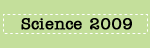•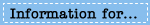•